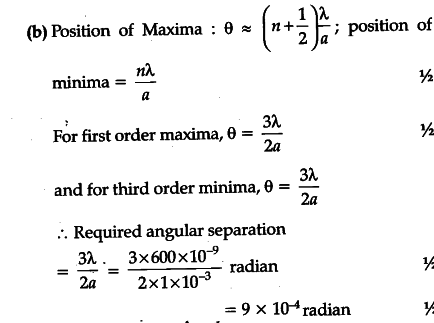# Write the factors by which the resolving power of a telescope can be increased

(a) Write the factors by which the resolving power of a telescope can be increased.
(b) Estimate the angular separation between first order maximum and third order minimum of the diffraction pattern due to a single slit of width 1 mm, when light of wavelength 600 nm is incident normal on it.

(a) The resolving power of a telescope, can be increased by
(i) increasing the diameter of its objective
(ii) using light of short wavelength
[Note : Give full credit even if a student writes just the first of these two factors.]